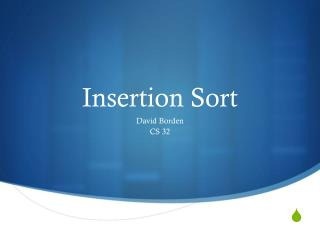DownloadDownload PresentationInsertion Sort

Insertion Sort

Télécharger la présentationInsertion Sort

- - - - - - - - - - - - - - - - - - - - - - - - - - - E N D - - - - - - - - - - - - - - - - - - - - - - - - - - -
Presentation Transcript

1. Insertion Sort David Borden CS 32

2. How Insertion Sort Works Somewhat how humans naturally sort things ( e.g. sorting books by alphabetical order ) http://commons.wikimedia.org/wiki/File:Insertion-sort-example-300px.gif Author: Swfung8

3. An element in the array is chosen every run-through of the sort • It is then inserted into the appropriately sorted place • This continues until everything is sorted • Which element is picked to be sorted first is a choice left to the implementer.

4. Sort { 7, -5, 2, 16, 4 } "Insertion Sort." INSERTION SORT (Java, C ). N.p., n.d. Web. 13 Aug. 2012. <http://www.algolist.net/Algorithms/Sorting/Insertion_sort>.

5. Sort { 7, -5, 2, 16, 4 }

6. Sort { 7, -5, 2, 16, 4 }

7. Sort { 7, -5, 2, 16, 4 }

8. Sort { 7, -5, 2, 16, 4 }

9. Sort { 7, -5, 2, 16, 4 }

10. Performance • Average Case: O( n2) • Random list • Worst Case: O( n2) • List in reverse order • Best Case: O ( n ) • (Almost) sorted list

11. Random Order http://www.sorting-algorithms.com/insertion-sort Author: 2007 David R. Martin.

12. Reverse order Notice how slow the sorting algorithm works in reverse order http://www.sorting-algorithms.com/insertion-sort Author: 2007 David R. Martin.

13. Properties • Stable: When sorting, elements with equal keys are not taken out of order • Adaptive: When the list is close to sorted, O( n ) • Very easy implementation http://www.sorting-algorithms.com/insertion-sort Author: 2007 David R. Martin.

14. In Practice • Used in practice only for small sets of data • It is the fastest sort for a small data set • Better than quicksort at a point • Other sorts sometimes use insert sort • Most efficient when used together • A faster spin-off of the insert sort is the Shell Sort • It is better for Shell Sort to be in a linked list or skip list, but the data structure does not matter for the Insertion Sort